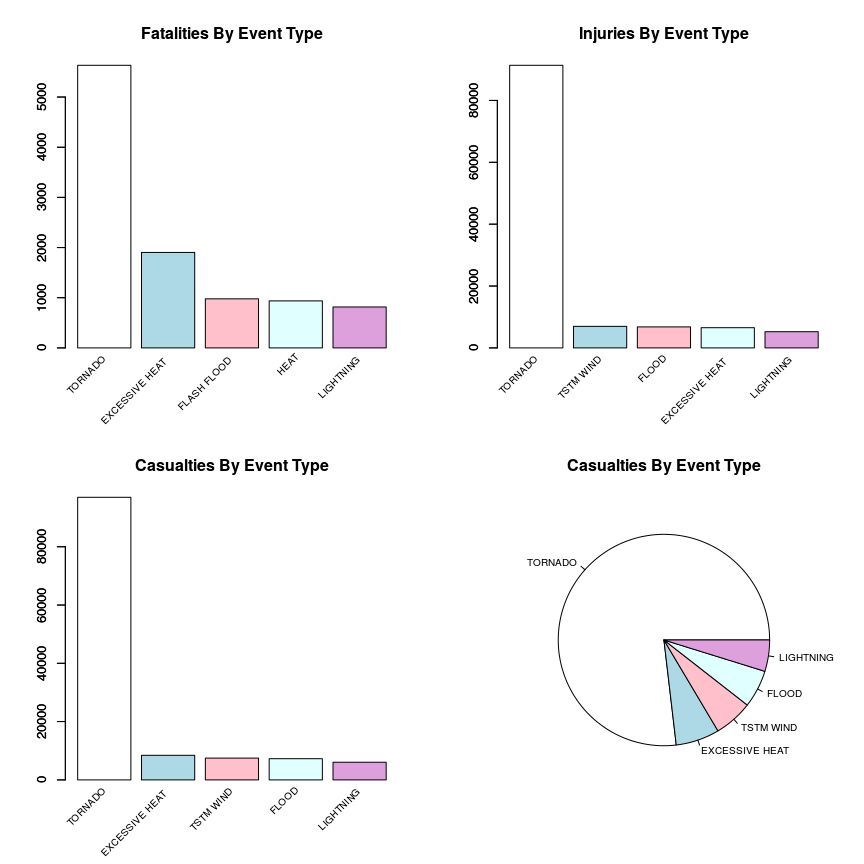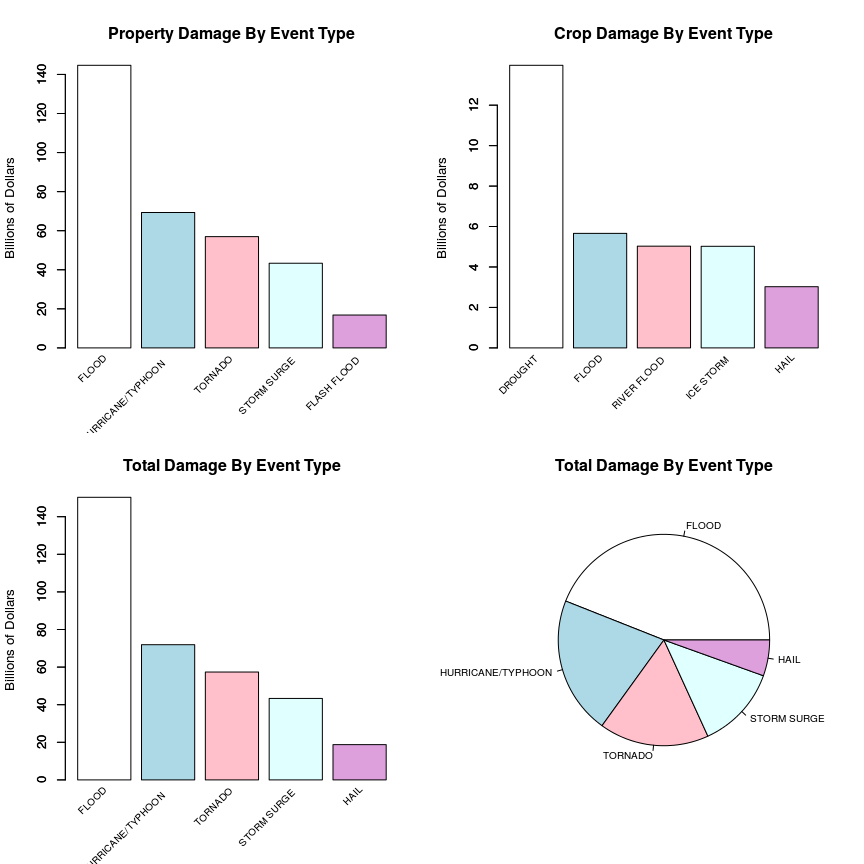## Synopsis

In this project we will perform a basic analysis of the effect of severe weather events, in both the public health and economic senses, on the community. For our analysis we will be using the U.S. National Oceanic and Atmospheric Administration’s (NOAA) storm database.

This database tracks characteristics of major storms and weather events in the United States, including when and where they occur, as well as estimates of any fatalities, injuries, and property damage.

We will attempt to answer the following questions:

1. Across the United States, which types of events are most harmful with respect to population health?

2. Across the United States, which types of events have the greatest economic consequences?

## Data Processing

First load the libraries required for the analysis.

    if (!require('reshape2')) {

install.packages('reshape2');
require('reshape2');
}
## Loading required package: reshape2

    if (!file.exists('./repdata-data-StormData.csv.bz2')) {

url = 'https://d396qusza40orc.cloudfront.net/repdata%2Fdata%2FStormData.csv.bz2',
destfile = './repdata-data-StormData.csv.bz2',
method = 'curl'
);
}

Next read the data, taking care to read it in as-is. The column names are listed below.

    storm_data <- read.csv(bzfile('repdata-data-StormData.csv.bz2'), as.is = TRUE);
names(storm_data);
##   "STATE__"    "BGN_DATE"   "BGN_TIME"   "TIME_ZONE"  "COUNTY"
##   "COUNTYNAME" "STATE"      "EVTYPE"     "BGN_RANGE"  "BGN_AZI"
##  "BGN_LOCATI" "END_DATE"   "END_TIME"   "COUNTY_END" "COUNTYENDN"
##  "END_RANGE"  "END_AZI"    "END_LOCATI" "LENGTH"     "WIDTH"
##  "F"          "MAG"        "FATALITIES" "INJURIES"   "PROPDMG"
##  "PROPDMGEXP" "CROPDMG"    "CROPDMGEXP" "WFO"        "STATEOFFIC"
##  "ZONENAMES"  "LATITUDE"   "LONGITUDE"  "LATITUDE_E" "LONGITUDE_"
##  "REMARKS"    "REFNUM"

Now that the data has been read in, begin the process of cleaning it. First, change the column names to lowercase, then retain only the columns that will be used in the analysis, and finally convert the event type column to a factor.

    names(storm_data) <- tolower(names(storm_data));
storm_data        <- storm_data[, c('evtype', 'fatalities', 'injuries', 'propdmg', 'propdmgexp', 'cropdmg', 'cropdmgexp')];
storm_data$evtype <- as.factor(storm_data$evtype);

We see that the casualty data is split across two columns - fatalities and injuries. Because of this, for the purpose of facilitating casualty analysis, I will add a column that combines the two columns into a total.

    storm_data$casualties <- storm_data$fatalities + storm_data$injuries; And taking a look at the cost data, we see each cost category is split into two - amount and exponent. Because of this, for the purpose of facilitating cost analysis, the two columns will be combined into a total, after converting the exponent into a numeric value. To achieve this we make use of a custom function (see below).  clean_numeric_data <- function(data) { data[data %in% c('', '+', '?', '-')] <- '0'; data[data %in% c('h', 'H')] <- '2'; data[data %in% c('k', 'K')] <- '3'; data[data %in% c('m', 'M')] <- '6'; data[data %in% c('b', 'B')] <- '9'; data <- as.numeric(data); return(data); } storm_data$propdmgexp <- clean_numeric_data(storm_data$propdmgexp); storm_data$cropdmgexp <- clean_numeric_data(storm_data$cropdmgexp); storm_data$propdmg    <- storm_data$propdmg * 10^storm_data$propdmgexp;
storm_data$cropdmg <- storm_data$cropdmg * 10^storm_data$cropdmgexp; storm_data$totaldmg   <- storm_data$propdmg + storm_data$cropdmg;

Now that the data has been put in a useful form we are in a position to proceed.

## Results

Now we address two questions with the help of some summaries and plots.

### Question 1: Across the United States, which types of events are most harmful with respect to population health?

Using melt and dcast functions from the reshape2 library we can calculate aggregate sums for both fatalities and injuries by event type, and in both cases we retain only the top 5 rows.

    fatalities_long <- melt(storm_data, id.vars = "evtype", measure.vars = "fatalities", na.rm = TRUE);
fatalities_wide <- dcast(fatalities_long, evtype ~ variable, sum);
fatalities      <- fatalities_wide[order(fatalities_wide$fatalities, decreasing = TRUE), ][1:5, ]; fatalities; ## evtype fatalities ## 830 TORNADO 5633 ## 123 EXCESSIVE HEAT 1903 ## 147 FLASH FLOOD 978 ## 269 HEAT 937 ## 452 LIGHTNING 816 We can see the top five severe weather event causes of fatalities above.  injuries_long <- melt(storm_data, id.vars = "evtype", measure.vars = "injuries", na.rm = TRUE); injuries_wide <- dcast(injuries_long, evtype ~ variable, sum); injuries <- injuries_wide[order(injuries_wide$injuries, decreasing = TRUE), ][1:5, ];

injuries;
##             evtype injuries
## 854      TSTM WIND     6957
## 164          FLOOD     6789
## 123 EXCESSIVE HEAT     6525
## 452      LIGHTNING     5230

And we can see the top five severe weather event causes of injuries above.

    casualties_long <- melt(storm_data, id.vars = "evtype", measure.vars = "casualties", na.rm = TRUE);
casualties_wide <- dcast(casualties_long, evtype ~ variable, sum);
casualties      <- casualties_wide[order(casualties_wide$casualties, decreasing = TRUE), ][1:5, ]; casualties; ## evtype casualties ## 830 TORNADO 96979 ## 123 EXCESSIVE HEAT 8428 ## 854 TSTM WIND 7461 ## 164 FLOOD 7259 ## 452 LIGHTNING 6046 And we can see the top five severe weather event causes of casualties above. Next we plot all of the above.  colors <- c('white', 'lightblue', 'pink', 'lightcyan', 'plum'); par(mfrow = c(2, 2)); fatalities_plot <- barplot( fatalities$fatalities,
main = 'Fatalities By Event Type',
col = colors
);
text(fatalities_plot, par('usr'), labels = fatalities$evtype, srt = 45, adj = c(1.1, 1.1), xpd = TRUE, cex = .75); axis(2); injuries_plot <- barplot( injuries$injuries,
main = 'Injuries By Event Type',
col = colors
);
text(injuries_plot, par('usr'), labels = injuries$evtype, srt = 45, adj = c(1.1, 1.1), xpd = TRUE, cex = .75); axis(2); casualties_plot <- barplot( casualties$casualties,
main = 'Casualties By Event Type',
col = colors
);
text(casualties_plot, par('usr'), labels = casualties$evtype, srt = 45, adj = c(1.1, 1.1), xpd = TRUE, cex = .75); axis(2); casualties_pie <- pie( casualties$casualties,
labels = casualties$evtype, main = 'Casualties By Event Type', cex = .75, col = colors );As we can see from the above plots, there is some consistency in the event type across fatalities and injuries - in fact three of the top five in both categories are consistent. ### Question 2: Across the United States, which types of events have the greatest economic consequences? Once again we use the melt and dcast functions from the reshape2 library to calculate aggregate sums of the cost of damage done to property, crops, and both by event type, and in both cases we retain only the top 5 rows.  propdmg_long <- melt(storm_data, id.vars = "evtype", measure.vars = "propdmg", na.rm = TRUE); propdmg_wide <- dcast(propdmg_long, evtype ~ variable, sum); propdmg <- propdmg_wide[order(propdmg_wide$propdmg, decreasing = TRUE), ][1:5, ];

propdmg;
##                evtype      propdmg
## 164             FLOOD 144657709807
## 406 HURRICANE/TYPHOON  69305840000
## 666       STORM SURGE  43323536000
## 147       FLASH FLOOD  16822673978

We can see the top five severe weather events ordered by cost of property damage above.

    cropdmg_long <- melt(storm_data, id.vars = "evtype", measure.vars = "cropdmg", na.rm = TRUE);
cropdmg_wide <- dcast(cropdmg_long, evtype ~ variable, sum);
cropdmg      <- cropdmg_wide[order(cropdmg_wide$cropdmg, decreasing = TRUE), ][1:5, ]; cropdmg; ## evtype cropdmg ## 88 DROUGHT 13972566000 ## 164 FLOOD 5661968450 ## 586 RIVER FLOOD 5029459000 ## 424 ICE STORM 5022113500 ## 238 HAIL 3025954473 And we can see the top five severe weather events ordered by cost of crop damage above.  totaldmg_long <- melt(storm_data, id.vars = "evtype", measure.vars = "totaldmg", na.rm = TRUE); totaldmg_wide <- dcast(totaldmg_long, evtype ~ variable, sum); totaldmg <- totaldmg_wide[order(totaldmg_wide$totaldmg, decreasing = TRUE), ][1:5, ];

totaldmg;
##                evtype     totaldmg
## 164             FLOOD 150319678257
## 406 HURRICANE/TYPHOON  71913712800
## 666       STORM SURGE  43323541000
## 238              HAIL  18761221986

And we can see the top five severe weather events ordered by cost of total damage above. Next we plot all of the above.

    par(mfrow = c(2, 2));

propdmg_plot <- barplot(
propdmg$propdmg/10^9, main = 'Property Damage By Event Type', ylab = 'Billions of Dollars', col = colors ); text(propdmg_plot, par('usr'), labels = propdmg$evtype, srt = 45, adj = c(1.1, 1.1), xpd = TRUE, cex = .75);
axis(2);

cropdmg_plot <- barplot(
cropdmg$cropdmg/10^9, main = 'Crop Damage By Event Type', ylab = 'Billions of Dollars', col = colors ); text(cropdmg_plot, par('usr'), labels = cropdmg$evtype, srt = 45, adj = c(1.1, 1.1), xpd = TRUE, cex = .75);
axis(2);

totaldmg_plot <- barplot(
totaldmg$totaldmg/10^9, main = 'Total Damage By Event Type', ylab = 'Billions of Dollars', col = colors ); text(totaldmg_plot, par('usr'), labels = totaldmg$evtype, srt = 45, adj = c(1.1, 1.1), xpd = TRUE, cex = .75);
axis(2);

totaldmg_pie <- pie(
totaldmg$totaldmg, labels = totaldmg$evtype,
main = 'Total Damage By Event Type',
cex = .75,
col = colors
);## Conclusions

From the above data analysis we can see that:

1. Tornados are most harmful with respect to population health with a total of 5633 fatalities and 91346 injuries
2. Floods have the greatest economic consequences in respect of damage to property with a total cost of $144,657,709,807 3. Droughts have the greatest economic consequences in respect of damage to crops with a total cost of$13,972,566,000

## Development Environment

    sessionInfo();
## R version 3.1.2 (2014-10-31)
## Platform: x86_64-pc-linux-gnu (64-bit)
##
## locale:
##   LC_CTYPE=en_US.UTF-8       LC_NUMERIC=C
##   LC_TIME=en_US.UTF-8        LC_COLLATE=en_US.UTF-8
##   LC_MONETARY=en_US.UTF-8    LC_MESSAGES=en_US.UTF-8
##   LC_PAPER=en_US.UTF-8       LC_NAME=C
##   rmarkdown_0.3.10 stringr_0.6.2    tools_3.1.2      yaml_2.1.11